Our Special Offer - Get 3 Courses at 24,999/- Only. Read more
Hire Talent (HR):+91-9707 240 250

# General# Java Operators

The operator is a symbol that says the compiler to perform explicit mathematical or logical manipulations.

### Types of Operators

• Unary Operators
• Binary Operators
• Ternary Operators

#### Unary operators

Unary operations have only one operand.

Example:

!      &        ~          *          ++        —        +          –

### Binary operators

The binary operators are operating on two operands. These operators can be arithmetic operators, relational, logical operators, bitwise, assignment operators.

### Arithmetic Operators

Example:

+      –       *      /        %

These operators are used in arithmetic expressions.

Example: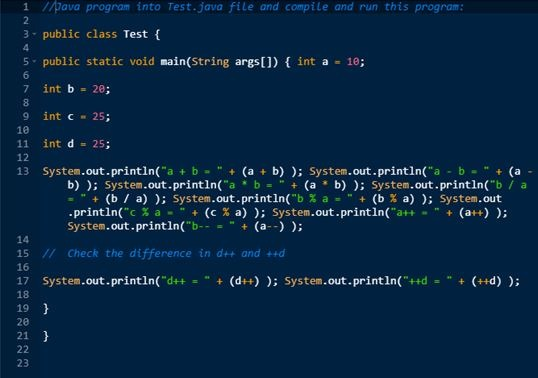Output: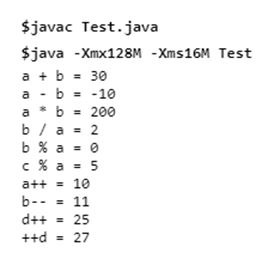### Relational Operators:

==       !=     >     <     >=       <=

These operators are used in relational operations. The relational operations always give 0 (or False) or 1 (or True).

Example:

Java program in Test.java file and compile and run this program. :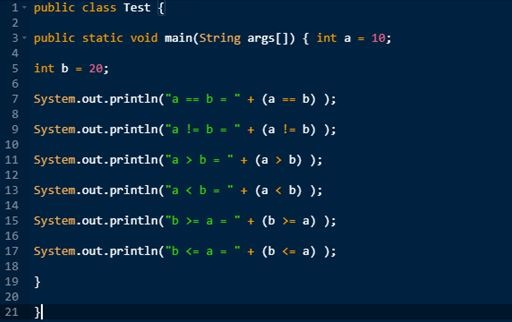Output: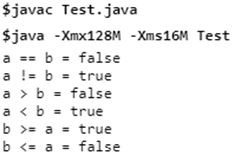### Logical Operators

The logical operators supported by Java

||   and  !

Logical operators are used in logical expressions. Such expressions always give 0 (or False) or 1 (or True).

Example: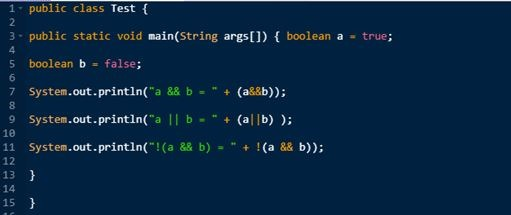Output: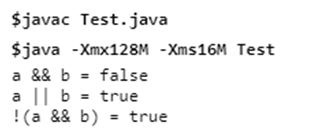### Bitwise Operators

Bitwise operator works on bits.

&         |       ^         ~          <<             >>

The Bitwise operators supported by C++ are listed in the following below table.

Example: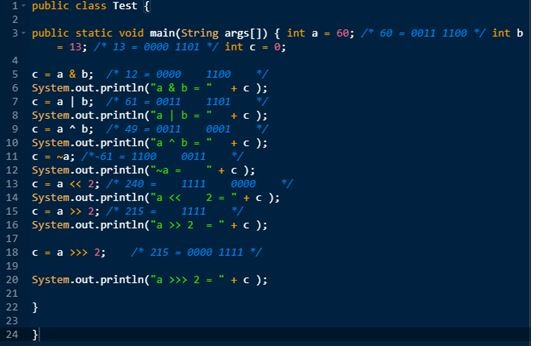Output: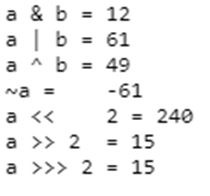### Shorthand operators

We combine the assignment operators with arithmetic operators and bitwise operators. Such operators are called as shorthand operators.

Example:

+    =   -=  * = /=  %=  &=  |=  ^=

### Assignment Operator

It is an operator used to assign a value to a variable.

Syntax:

variable = value or expression;
int  a=5;

### Special Operators

Some special operators are there in C++.

Example:

sizeof()     comma(,)      dot(.)    pointer(*)

### Ternary operators or conditional operators

The ternary operators are those operators that operate on three or more operands. Ternary operator is also called as conditional operator. This operator can be used as an alternative to if-else statement.

#### Precedence of operators or Hierarchy of operators

If an expression contains multiple operators, the order in which operations are to be carried out is called hierarchy.

Example:

x = 3 + 1 * 5;

Here x is assigned 08, not 20 because operator * has higher precedence than +. First, multiply 1 and 5 and then adds into 3 and assign it to the variable x.

### InstanceOf Operator

The instanceOf operator is wriiten as:

( Object reference variable )    instanceOf         (class/interface type)

### Precedence of Java Operators:

 Category Operator Associativity Postfix () [] . (dot operator) Left to right Unary ++ – – ! ~ Right to left Multiplicative * / % Left to right Additive + – Left to right Shift >> >>> << Left to right Relational > >= < <= Left to right Equality == != Left to right Bitwise AND & Left to right